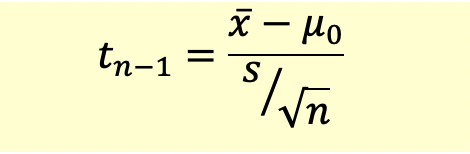# Hypothesis Testing with t-statistic

t-statistic is the computed value of the test statistic based on the Student’s t distribution.

t-statistic with n-1 degrees of freedom is calculated as follows:In the hypothesis test, we compare the t-statistic with the critical t-value at a given level of significance and appropriate degrees of freedom.